# SSAT Upper Level Math : SSAT Upper Level Quantitative (Math)

## Example Questions

### Example Question #1 : How To Find The Frequency Of An Event

In a small school, ninth grade comprises 54 students, including 22 boys. Each student is assigned to one of two home rooms - those of Mr. Brooks and Mrs. Kelly. The boys and the girls are both divided evenly between the home rooms.

According to the charter, two of the student council representatives from the ninth grade will be selected by choosing one at random from each home room. What is the probability that both representatives selected will be girls?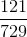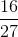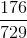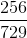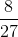Explanation:

22 out of the 54 students are boys, so 32 are girls. Also, since the boys and the girls are split evenly between the home rooms, each home room has 11 boys and 16 girls for a total of 27 students.

This means that the probability of a randomly selected student from Mr. Brooks's home room being a girl is; the probability is the same for Mrs. Kelly's homeroom. By the multiplication principle, the probability of both students being girls is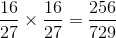### Example Question #2 : How To Find The Frequency Of An Event

Three fair six-sided dice are rolled, and the numbers are multiplied. What are the odds against rolling an odd product?

8 to 1

7 to 1

4 to 1

2 to 1

Even

7 to 1

Explanation:

Three whole numbers have an odd product if and only if all three factors are odd. Therefore, for three dice to show an odd product, all three dice must show odd numbers.

The probability of a die showing an odd number is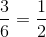, so the probability of all three dice showing odd numbers - equivalently, the probability of an odd product - is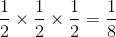. This translates to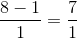or 7 to 1 odds against an odd product.

### Example Question #3 : How To Find The Frequency Of An Event

Three fair six-sided dice are rolled, and the numbers are added. What is the probability that the sum will be 16 or greater?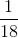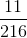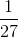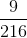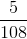Explanation:

There are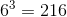outcomes for the roll of three six-sided dice. Of these outcomes, the following are the ways to roll a 16 or greater: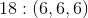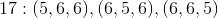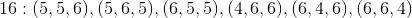This is 10 rolls with the desired outcome out of a possible 216, which translates to a probability of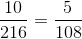### Example Question #4 : How To Find The Frequency Of An Event

The aces, jacks, kings, and queens are removed from a red-backed deck of standard playing cards; the same is done with a blue-backed deck. One card is removed at random from each deck; the numbers are multiplied together. What are the odds of the product being odd?

The correct answer is not given among the other responses.

Even

5 to 4 against

7 to 2 against

3 to 1 against

The correct answer is not given among the other responses.

Explanation:

Two whole numbers have an odd product if and only if both factors are odd.

After the aces and face cards are removed from each deck, there are nine ranks left - the twos through tens - each of which are represented by four cards and each of which are therefore equally probable in each deck. The probability of drawing an odd card from the red deck is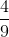, and the same holds for the blue deck. The probability of drawing two odd cards  - equivalently, the probability of an odd product - is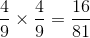; this translates to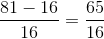or 65 to 16 odds against this occurrence. This answer is not among the responses.

### Example Question #5 : How To Find The Frequency Of An Event

In a small school, ninth grade comprises 50 students, including 18 boys. Each student is assigned to one of two home rooms - those of Mr. Jones and Mrs. Wilson. The boys and the girls are both divided evenly between the home rooms.

According to the charter, two of the student council representatives from the ninth grade will be selected by choosing one at random from each home room. What are the odds of one boy and one girl being selected?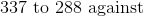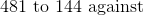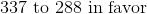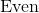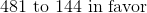Explanation:

18 out of the 50 students are boys, so 32 are girls. Also, since the boys and the girls are split evenly between the home rooms, each home room has 9 boys and 16 girls for a total of 25 students.

This means that the probability of a randomly selected student from Mr. Jones's home room being a boy is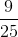, and that of the student being a girl is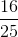. These probabilities are the same for Mrs. Wilson's class.

Therefore, the probability of one boy being selected from Mr. Jones's class and one girl being selected from Mrs. Wilson's class is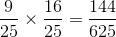; the probability of the reverse event is the same. Therefore, the probability of one boy and one girl being selected is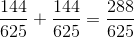The odds against this happening are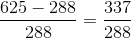- that is, 337 to 288.

### Example Question #6 : How To Find The Frequency Of An Event

If Bob rolled 3 fair six sided dice, what is the probability of NOT rolling a sum of 3?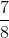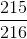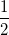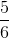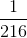Explanation:

The only possible combination to roll a sum of 3 is by rolling three 1s.  The probability of rolling any side of a die is.

The odds of rolling three 1s are: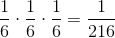Therefore, the probability of NOT rolling three 1s is: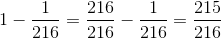### Example Question #1 : Outcomes

A fair coin is tossed nine times. Each toss comes up heads. What is the probability that the coin will come up heads a tenth time?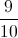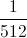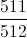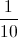Explanation:

Each flip of the coin is an independent event, and does not affect the other flips; all that matters is that the coin is fair. Because of that fact, the probability that the tenth flip comes up heads is.

### Example Question #2 : Outcomes

Ten balls - five green, three blue, two yellow - are placed in a hat. Two balls are drawn, one after the other, without replacement. What is the probability that at least one will be green?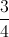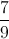Explanation:

This can more easily be solved by finding the probability that neither will be green, then subtracting it from 1.

The probability that the first ball will be not be green is five out of ten, or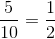.

This will leave nine balls, four not green, making the probability that the second ball will then not be green.

Multiply these probabilities: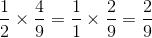This is the probability that neither ball is green; subtract this from 1: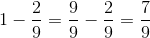### Example Question #3 : Outcomes

Six boxes are marked with numbers 1 to 6. Each box contains ten marbles, with the number of red marbles in each box corresponding to its number, and the remainder being white.

A fair die is rolled. The box corresponding to the roll is chosen and a marble is drawn at random from that box. What is the probability of drawing a red marble?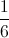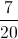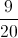Explanation:

The die is fair, making each box equally likely to be chosen; also, each marble is equally likely to be chosen after the box is chosen. This makes each marble overall equally likely to be chosen. Since there are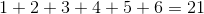red marbles out of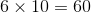marbles total, the probability of choosing a red marble is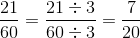### Example Question #4 : Outcomes

Six boxes are marked with numbers 1 to 6. Each box contains ten marbles, with the number of red marbles in each box corresponding to its number, and the remainder being white.

A fair die is rolled. The box corresponding to the roll is chosen and a marble is drawn at random from that box. What is the probability of drawing a white marble?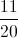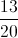Explanation:

The die is fair, making each box equally likely to be chosen; also, each marble is equally likely to be chosen after the box is chosen. This makes each marble overall equally likely to be chosen. Since there are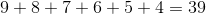white marbles out ofmarbles total, the probability of choosing a white marble is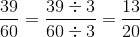### All SSAT Upper Level Math Resources